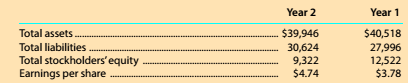Chapter 8, Problem 8.8.5MBA

Chapter
Section
Textbook Problem

Debt and price-earnings ratios The Home Depot. Inc. (HD) operates over 2.200 home improvement retail stores and is a competitor of Lowe's (LOW). The following data (in millions) were adapted from recent financial statements of The Home Depot.Comparing Years 1 and 2, should creditors feel more or less safe in Year 2?

To determine

Concept Introduction:

Debt Ratio:

Debt ratio is the relationship between the Total liabilities and Total Assets of a corporation. Debt ratio shows the part of assets financed by debts. It is calculated by dividing total liabilities by total assets. The formula of debt ratio is as follows:

Debt Ratio = Total LiabilitiesTotal Assets

To Indicate:

The Safety for creditors in year 2 as compared with year 2

Explanation

The Debt Ratios for year 1 and 2 are calculated as follows:

 $in Millions Year 1 Year 2 Total Liabilities (A)$30,624 \$27,996

Still sussing out bartleby?

Check out a sample textbook solution.

See a sample solution

The Solution to Your Study Problems

Bartleby provides explanations to thousands of textbook problems written by our experts, many with advanced degrees!

Get Started